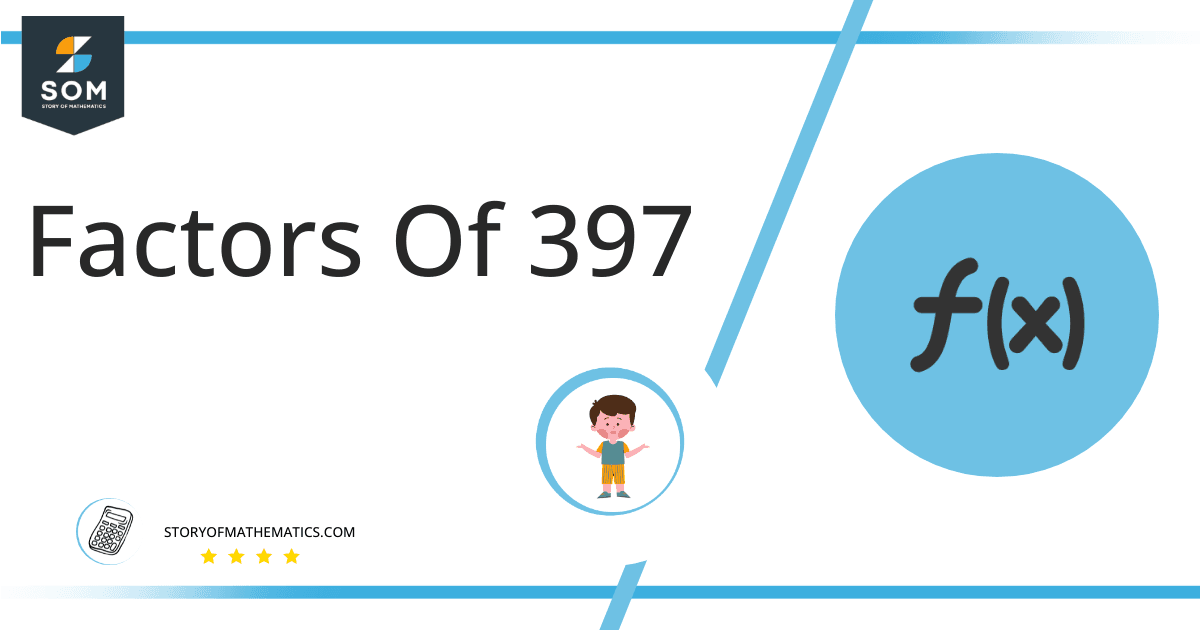# Factors of 397 : Prime Factorization, Methods, and Examples

A group of numbers known as the factors of 397 produces no remainder when 397 is divided by them. A factor cannot exceed a number, although it may be less than or equal.Any two integer factors can be multiplied to get the number; these factors couple together to form factor pairs.

### Factors of 397

Here are the factors of number 397.

Factors of 397: 1 and 397

### Negative Factors of 397

The negative factors of 397  are similar to their positive aspects, just with a negative sign.

Negative Factors of 397 : -1 and -397

### Prime Factorization of 397

The prime factorization of 397  is the way of expressing its prime factors in the product form.

Prime Factorization: 397

In this article, we will learn about the factors of 397 and how to find them using various techniques such as upside-down division, prime factorization, and factor tree.

## What Are the Factors of 397?

The factors of 397 are1 and 397. These numbers are the factors as they do not leave any remainder when divided by 397.

The factors of 397  are classified as prime numbers and composite numbers. The prime factors of the number 397 can be determined using the prime factorization technique.

## How To Find the Factors of 397?

You can find the factors of 397  by using the rules of divisibility. The divisibility rule states that any number, when divided by any other natural number, is said to be divisible by the number if the quotient is the whole number and the resulting remainder is zero.

To find the factors of 397, create a list containing the numbers that are exactly divisible by 397 with zero remainders. One important thing to note is that 1 and 397 are the 397 ‘s factors as every natural number has 1 and the number itself as its factor.

1 is also called the universal factor of every number. The factors of 397 are determined as follows:

$\dfrac{397 }{1} = 397$

$\dfrac{397 }{397 } = 1$

Therefore, 1 and 397 are the factors of 397.

### Total Number of Factors of 397

For 397, there are 2 positive factors and 2 negative ones. So in total, there are 4 factors of 397.

To find the total number of factors of the given number, follow the procedure mentioned below:

1. Find the factorization/prime factorization of the given number.
2. Demonstrate the prime factorization of the number in the form of exponent form.
3. Add 1 to each of the exponents of the prime factor.
4. Now, multiply the resulting exponents together. This obtained product is equivalent to the total number of factors of the given number.

By following this procedure, the total number of factors of 397 is given as:

Factorization of 397 is 1 and 397.

The exponent of 1 and 397 is 1.

Adding 1 to each and multiplying them together results in m.

Therefore, the total number of factors of 397 is 4. 2 are positive, and 2 factors are negative.

### Important Notes

Here are some essential points that must be considered while finding the factors of any given number:

• The factor of any given number must be a whole number.
• The factors of the number cannot be in the form of decimals or fractions.
• Factors can be positive as well as negative.
• Negative factors are the additive inverse of the positive factors of a given number.
• The factor of a number cannot be greater than that number.
• Every even number has 2 as its prime factor, the smallest prime factor.

## Factors of 397 by Prime Factorization

The number 397  is a  prime number. Prime factorization is a valuable technique for finding the number’s prime factors and expressing the number as the product of its prime factors.Before finding the factors of 397 using prime factorization, let us find out what prime factors are. Prime factors are the factors of any given number that are only divisible by 1 and themselves.

To start the prime factorization of 397, start dividing by its most minor prime factor. First, determine that the given number is either even or odd. If it is an even number, then 2 will be the smallest prime factor.

Continue splitting the quotient obtained until 1 is received as the quotient. The prime factorization of 397  can be expressed as:

397  = 1 x 397

## Factors of 397 in Pairs

The factor pairs are the duplet of numbers that, when multiplied together, result in the factorized number. Factor pairs can be more than one depending on the total number of factors.For 397, the factor pairs can be found as:

1 x 397  = 397

The possible factor pairs of 397 are given as (1, 397 ).

All these numbers in pairs, when multiplied, give X as the product.

The negative factor pairs of 397 are given as:

-1 x -397 = 397

It is important to note that in negative factor pairs, the minus sign has been multiplied by the minus sign, due to which the resulting product is the original positive number. Therefore, -1 and -397 are called negative factors of 397.

The list of all the factors of 397, including positive and negative numbers, is given below.

Factor list of 397 : 1, -1, 397 , and -397

## Factors of 397 Solved Examples

To better understand the concept of factors, let’s solve some examples.

### Example 1

How many factors of 397  are there?

### Solution

The total number of Factors of 397 is 4.

Factors of 397 are 1 and 397.

### Example 2

Find the factors of 397  using prime factorization.

### Solution

The prime factorization of 397 is given as:

397 $\div$ 397 = 1

So the prime factorization of 397 can be written as:

1 x 397 = 397